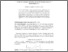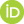# Statements and open problems on decidable sets X⊆N that contain informal notions and refer to the current knowledge on X

Kozdęba, Agnieszka and Tyszka, Apoloniusz (2022) Statements and open problems on decidable sets X⊆N that contain informal notions and refer to the current knowledge on X. [Preprint]Preview
Text
kozdeba_tyszka.pdf

## Abstract

Let f(1)=2, f(2)=4, and let f(n+1)=f(n)! for every integer n≥2. Edmund Landau's conjecture states that the set P(n^2+1) of primes of the form n^2+1 is infinite. Landau's conjecture implies the following unproven statement Φ: card(P(n^2+1))<ω ⇒ P(n^2+1)⊆[2,(((24!)!)!)!]. Let B denote the system of equations: {x_j!=x_k: j,k∈{1,...,9}}∪{x_i⋅x_j=x_k: i,j,k∈{1,...,9}}. We write some system U⊆B of 9 equations which has exactly two solutions in positive integers x_1,...,x_9, namely (1,...,1) and (f(1),...,f(9)). No known system S⊆B with a finite number of solutions in positive integers x_1,...,x_9 has a solution (x_1,...,x_9)∈(N\{0})^9 satisfying max(x_1,...,x_9)>f(9). For every known system S⊆B, if the finiteness/infiniteness of the set {(x_1,...,x_9)∈(N\{0})^9: (x_1,...,x_9) solves S} is unknown, then the statement ∃ x_1,...,x_9∈N\{0} ((x_1,...,x_9) solves S)∧(max(x_1,...,x_9)>f(9)) remains unproven. We write some system A⊆B of 8 equations. Let Λ denote the statement: if the system A has at most finitely many solutions in positive integers x_1,...,x_9, then each such solution (x_1,...,x_9) satisfies x_1,...,x_9≤f(9). The statement Λ is equivalent to the statement Φ. It heuristically justifies the statement Φ . This justification does not yield the finiteness/infiniteness of P(n^2+1). We present a new heuristic argument for the infiniteness of P(n^2+1), which is not based on the statement Φ. Algorithms always terminate. The next statements and open problems justify the title of the article and involve epistemic and informal notions. We explain the distinction between existing algorithms (i.e. algorithms whose existence is provable in ZFC) and known algorithms (i.e. algorithms whose definition is constructive and currently known). Assuming that the infiniteness of a set X⊆N is false or unproven, we define which elements of X are classified as known. No known set X⊆N satisfies Conditions (1)-(4) and is widely known in number theory or naturally defined, where this term has only informal meaning. *** (1) A known algorithm with no input returns an integer n satisfying card(X)<ω ⇒ X⊆(-∞,n]. (2) A known algorithm for every k∈N decides whether or not k∈X. (3) No known algorithm with no input returns the logical value of the statement card(X)=ω. (4) There are many elements of X and it is conjectured, though so far unproven, that X is infinite. (5) X is naturally defined. The infiniteness of X is false or unproven. X has the simplest definition among known sets Y⊆N with the same set of known elements. *** Conditions (2)-(5) hold for X=P(n^2+1). The statement Φ implies Condition (1) for X=P(n^2+1). We define a set X⊆N which satisfies Conditions (1)-(5) except the requirement that X is naturally defined. The conjecture that there are infinitely many primes of the form k!+1 implies that the set N \setminus X is finite. We present a table that shows satisfiable conjunctions of the form #(Condition 1) ∧ (Condition 2) ∧ #(Condition 3) ∧ (Condition 4) ∧ #(Condition 5), where # denotes the negation ¬ or the absence of any symbol. No set X⊆N will satisfy Conditions (1)-(4) forever, if for every algorithm with no input, at some future day, a computer will be able to execute this algorithm in 1 second or less. The physical limits of computation disprove this assumption. The article was presented at the 25th Conference Applications of Logic in Philosophy and the Foundations of Mathematics, http://www.applications-of-logic.uni.wroc.pl/Program-1

 Export/Citation: EndNote | BibTeX | Dublin Core | ASCII/Text Citation (Chicago) | HTML Citation | OpenURL
 Social Networking:

Item Type: Preprint
Creators:
CreatorsEmailORCIDKozdęba, Agnieszkaagnieszka.kozdeba@gmail.com0000-0003-1770-375X
Tyszka, Apoloniuszrttyszka@cyf-kr.edu.pl0000-0002-2770-5495
Keywords: algorithms whose existence is provable in ZFC, conjecturally infinite sets X⊆N, constructively defined integer n satisfies card(X)<ω ⇒ X⊆(-∞,n], current knowledge on a set X⊆N, known elements of a set X⊆N whose infiniteness is false or unproven, mathematical definitions, theorems and open problems with epistemic and informal notions, physical limits of computation, primes of the form n^2+1, primes of the form n!+1, X is decidable by a constructively defined algorithm which is currently known
Subjects: Specific Sciences > Mathematics > Foundations
Specific Sciences > Mathematics > Logic
Specific Sciences > Computation/Information
Depositing User: Apoloniusz Tyszka
Date Deposited: 24 Aug 2022 21:52
Item ID: 21069
Subjects: Specific Sciences > Mathematics > Foundations
Specific Sciences > Mathematics > Logic
Specific Sciences > Computation/Information
Date: 3 January 2022
URI: http://philsci-archive.pitt.edu/id/eprint/21069

### Monthly Views for the past 3 yearsView Item